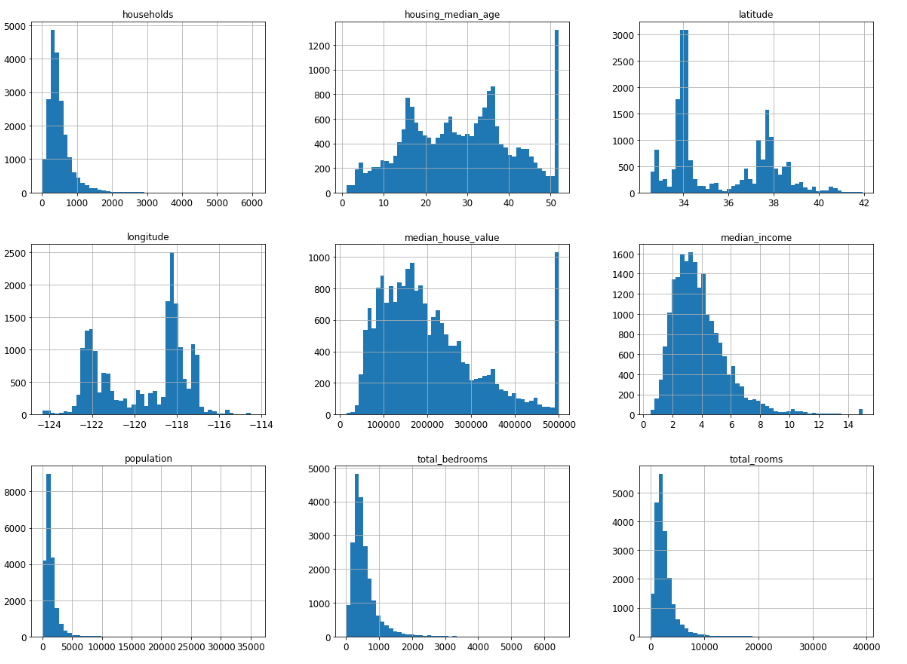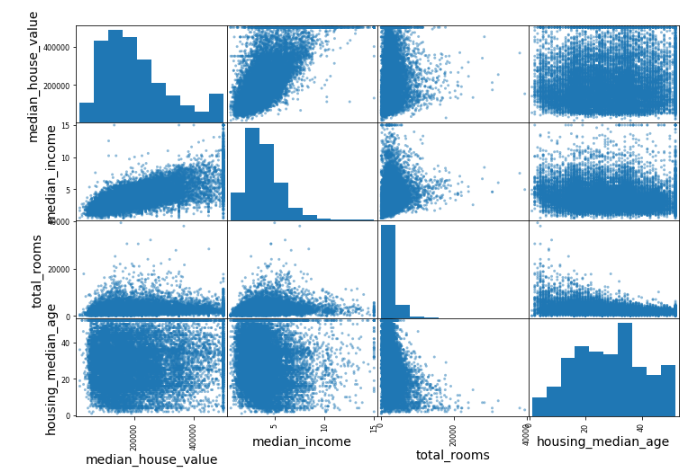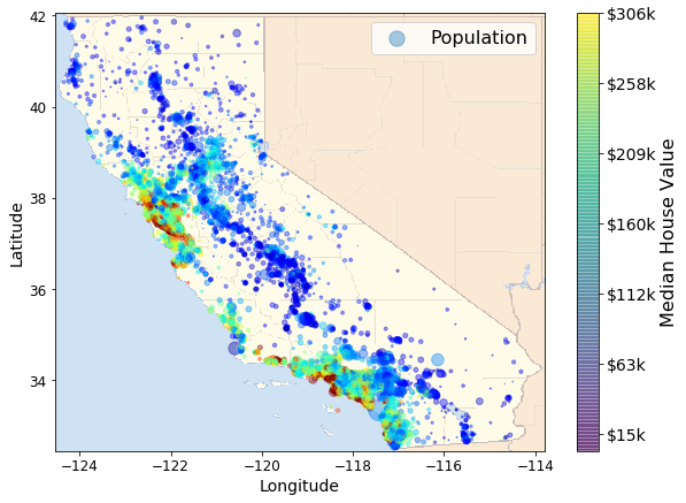# Summary of Chapter 2, End-to-End Machine Learning Project

This article summaries machine learning process in plain languages. Surprisingly, the most important isn’t the fansy modelling…

Personally, I split the understanding on machine learning into 3 levels.

1. Just for understanding - Just the concept and idea
2. Techician - Use of library and tools to build machine learning project
3. Scientist/Engineer - Understand the maths and statistics behind the models.

This article summarizes the machine learning process in plain language as much as posible. It covers the general concepts and gives you rough ideas of how does machine learning work, leaving the deep stuffs untouched. So this summary is great for the first level, also serves a good start of the next two levels.

For details and source code, please refer to the reference. Based on individuals background and time available, there are various versions to you can choose to read, 1-minute-summary, 10-minutes-summary.

## 1-minute-summary

If you want to conclude machine learning project within 5 steps, here is how I do it.

1. Discover and visualize the data to gain insights.
2. Prepare the data for Machine Learning algorithms.
3. Select a model and train it.

As you can probably tell, training a model is not the main part of machine learning. In fact, even though building machine learning models is the core, it is only a smart part of the whole machine learning project. I had this mis-understanding before I read this book. That’s also the most important lesson I learn from Chapter 2.

## 10-minutes summary

### First step, understand your data

It is important to understand your data. And the most intuitive way is to visualize it.

#### For individual features

Use histogram to plot distribution of individual features. The distribution can be useful. For example, you can obtain the support(range) of the feature. Sometimes, it is important to stratify the data and make sure each class has approximately same amount of training data.

housing.hist(bins=50, figsize=(20,15))
plt.show()#### Feature correlations

A quick and efficient way to understand how “related” a feature with the goal is to draw the coorelations.

scatter_matrix(housing[attributes], figsize=(12, 8))#### Geographical

If the data has geographical or geometric nature, plotting against the nature shape of the data will be very helpful too.### Secondly, prepare the data

Just like a chef needs to prepare the food before cooking, a data analyst needs to prepare the data before modeling. Common approaches include: (1) fill missing values in raw data (2) derive additional features (3) categorize data (4) standardization and normalization (4) others

### Next, modeling

#### Try many models

Most of the machine learning problems can be treated as either classification problems or regression problems. There are many models for each category. For example, for classification problem, there are SVM, Decision Tree, Random Forest, Logistic Regression, Native Bayes, etc. Based on the nature of the problem and the data, some models fit better, some poor. So try out multiple models to your problem and select the few that fit better.

Prediction result can be improved by “ensemble method”, which will be discussed in the future chapters.

#### Model Evaluation**

The accurary of a model can be evaluated using a technique called cross validation.

Intuitively, a model of high accuracy seems to be better. That’s a good guess, but not a complete answer. Based on the nature of the problems, especially the cost of wrong predictions, accuracy is not the only benchmark.

### Last but not least, Fine-tune the model, and Launch

There are probably a few hyper-parameters in yours models. Certain methods like randomized search can be used to find the optimal parameters. Also, ensemble methods may give you better overall results.

It is fun to try and build models. And it is more meaningful to finally put it in the real environment. However, you may need to regularly re-train your model.

### Reference

This article is part of a series of summaries on the book Hands-On Machine Learning with Scikit-Learn and TensorFlow from O’Reilly. The source codes are from either the book itself or this GitHub repository https://github.com/ageron/handson-ml.

I strongly recommend this book for machine learning beginners. It introduces the machine learning with two of the most popular machine learning tools, TensorFlow and Scikit-Learn. What’s more, it does not require deep maths and programming knowledge as pre-requisites. All you need to know is the very basic of Python.

Written on March 20, 2018